SNOSAW8E May   2008  – September 2015

PRODUCTION DATA.

1. Features
2. Applications
3. Description
4. Revision History
5. Description continued
6. Pin Configuration and Functions
7. Specifications
8. Detailed Description
1. 8.1 Overview
2. 8.2 Functional Block Diagram
3. 8.3 Feature Description
4. 8.4 Device Functional Modes
9. Application and Implementation
1. 9.1 Application Information
2. 9.2 Typical Application
10. 10Power Supply Recommendations
11. 11Layout
12. 12Device and Documentation Support
13. 13Mechanical, Packaging, and Orderable Information

• DBV|5

## 7 Specifications

### 7.1 Absolute Maximum Ratings

over operating free-air temperature range (unless otherwise noted)(1)(4)
MIN MAX UNIT
VIN Differential ±10 V
Output Short Circuit Current See (2)
Supply Voltage (VS = V+ - V) 35 V
Voltage at Input/Output pins V+ + 0.8 V − 0.8 V
Junction Temperature(3) 150 °C
Soldering Information:    Infrared or Convection (20 sec.) 235 °C
Wave Soldering (10 sec.) 260 °C
Storage Temperature −65 150 °C
(1) Stresses beyond those listed under Absolute Maximum Ratings may cause permanent damage to the device. These are stress ratings only, which do not imply functional operation of the device at these or any other conditions beyond those indicated under Recommended Operating Conditions. Exposure to absolute-maximum-rated conditions for extended periods may affect device reliability.
(2) Applies to both single-supply and split-supply operation. Continuous short circuit operation at elevated ambient temperature can result in exceeding the maximum allowed junction temperature of 150°C. Short circuit test is a momentary test. Output short circuit duration is infinite for VS ≤ 6V at room temperature and below. For VS > 6V, allowable short circuit duration is 1.5 ms.
(3) The maximum power dissipation is a function of TJ(MAX), RθJA. The maximum allowable power dissipation at any ambient temperature is PD = (TJ(MAX)) – TA)/ RθJA. All numbers apply for packages soldered directly onto a PCB.
(4) If Military/Aerospace specified devices are required, please contact the Texas Instruments Sales Office/Distributors for availability and specifications.

### 7.2 ESD Ratings

VALUE UNIT
V(ESD) Electrostatic discharge(3) Human body model (HBM), per ANSI/ESDA/JEDEC JS-001(1) ±2000 V
Charged-device model (CDM), per JEDEC specification JESD22-C101(2) ±1000
Machine Model 200
(1) JEDEC document JEP155 states that 500-V HBM allows safe manufacturing with a standard ESD control process.
(2) JEDEC document JEP157 states that 250-V CDM allows safe manufacturing with a standard ESD control process.
(3) Human Body Model, applicable std. MIL-STD-883, Method 3015.7. Machine Model, applicable std. JESD22-A115-A (ESD MM std. of JEDEC)Field-Induced Charge-Device Model, applicable std. JESD22-C101-C (ESD FICDM std. of JEDEC).

### 7.3 Recommended Operating Conditions

MIN MAX UNIT
Supply Voltage (VS = V+ - V) 2.5 32 V
Temperature Range(1) −40 125 °C
(1) The maximum power dissipation is a function of TJ(MAX), RθJA. The maximum allowable power dissipation at any ambient temperature is PD = (TJ(MAX)) – TA)/ RθJA. All numbers apply for packages soldered directly onto a PCB.

### 7.4 Thermal Information

THERMAL METRIC(2) LM7321 UNIT
D (SOIC) DBV (SOT) DGK (VSSOP)
8 PINS 5 PINS 8 PINS
RθJA(1)      Junction-to-ambient thermal resistance 165 325 235 °C/W
(1) The maximum power dissipation is a function of TJ(MAX), RθJA. The maximum allowable power dissipation at any ambient temperature is PD = (TJ(MAX)) – TA)/ RθJA. All numbers apply for packages soldered directly onto a PCB.

### 7.5 2.7-V Electrical Characteristics

Unless otherwise specified, all limits ensured for TA = 25°C, V+ = 2.7 V, V = 0 V, VCM = 0.5 V, VOUT = 1.35 V, and RL > 1 MΩ to 1.35 V.(1)
PARAMETER TEST CONDITION MIN(3) TYP(2) MAX(3) UNIT
VOS Input Offset Voltage VCM = 0.5 V and VCM = 2.2 V −5 ±0.7 +5 mV
TA = –40°C to +125°C −6 +6
TC VOS Input Offset Voltage Temperature Drift VCM = 0.5 V and VCM = 2.2 V(4) ±2 µV/C
IB Input Bias Current VCM = 0.5 V(5) −2 −1.2 µA
TA = –40°C to +125°C −2.5
VCM = 2.2 V(5) 0.45 1
TA = –40°C to +125°C 1.5
IOS Input Offset Current VCM = 0.5 V and VCM = 2.2 V 20 200 nA
TA = –40°C to +125°C 300
CMRR Common-Mode Rejection Ratio 0 V ≤ VCM ≤ 1 V 70 100 dB
TA = –40°C to +125°C 60
0 V ≤ VCM ≤ 2.7 V 55 70
TA = –40°C to +125°C 50
PSRR Power Supply Rejection Ratio 2.7 V ≤ VS ≤ 30 V 78 104 dB
TA = –40°C to +125°C 74
CMVR Common-Mode Voltage Range (Min) CMRR > 50 dB −0.3 −0.1 V
TA = –40°C to +125°C 0
Common-Mode Voltage Range (Max) CMRR > 50 dB 2.8 3
TA = –40°C to +125°C 2.7
AVOL Open-Loop Voltage Gain 0.5 V ≤ VO ≤ 2.2 V
RL = 10 kΩ to 1.35 V
65 72 dB
TA = –40°C to +125°C 62
0.5 V ≤ VO ≤ 2.2 V
RL = 2 kΩ to 1.35V
59 66
TA = –40°C to +125°C 55
VOUT Output Voltage Swing
High
RL = 10 kΩ to 1.35 V
VID = 100 mV
50 150 mV from either rail
TA = –40°C to +125°C 160
RL = 2 kΩ to 1.35 V
VID = 100 mV
100 250
TA = –40°C to +125°C 280
Output Voltage Swing
Low
RL = 10 kΩ to 1.35 V
VID = −100 mV
20 120
TA = –40°C to +125°C 150
RL = 2 kΩ to 1.35 V
VID = −100 mV
40 120
TA = –40°C to +125°C 150
IOUT Output Current Sourcing
VID = 200 mV, VOUT = 0 V(7)
30 48 mA
TA = –40°C to +125°C 20
Sinking
VID = −200 mV, VOUT = 2.7 V(7)
40 65
TA = –40°C to +125°C 30
IS Supply Current LM7321 0.95 1.3 mA
TA = –40°C to +125°C 1.9
LM7322 2 2.5
TA = –40°C to +125°C 3.8
SR Slew Rate(6) AV = +1, VI = 2-V Step 8.5 V/µs
fu Unity Gain Frequency RL = 2 kΩ, CL = 20 pF 7.5 MHz
GBW Gain Bandwidth f = 50 kHz 16 MHz
en Input Referred Voltage Noise Density f = 2 kHz 11.9 nV/√H
in Input Referred Current Noise Density f = 2 kHz 0.5 pA/√H
THD+N Total Harmonic Distortion + Noise V+ = 1.9 V, V = −0.8 V
f = 1 kHz, RL = 100 kΩ, AV = +2
VOUT = 210 mVPP
−77 dB
CT Rej. Crosstalk Rejection f = 100 kHz, Driver RL = 10 kΩ 60 dB
(1) Electrical Table values apply only for factory testing conditions at the temperature indicated. Factory testing conditions result in very limited self-heating of the device such that TJ = TA. No ensured specification of parametric performance is indicated in the electrical tables under conditions of internal self-heating where TJ > TA.
(2) Typical values represent the most likely parametric norm as determined at the time of characterization. Actual typical values may vary over time and will also depend on the application and configuration. The typical values are not tested and are not ensured on shipped production material.
(3) All limits are ensured by testing or statistical analysis.
(4) Offset voltage temperature drift determined by dividing the change in VOS at temperature extremes into the total temperature change.
(5) Positive current corresponds to current flowing into the device.
(6) Slew rate is the slower of the rising and falling slew rates. Connected as a Voltage Follower.
(7) Applies to both single-supply and split-supply operation. Continuous short circuit operation at elevated ambient temperature can result in exceeding the maximum allowed junction temperature of 150°C. Short circuit test is a momentary test. Output short circuit duration is infinite for VS ≤ 6 V at room temperature and below. For VS > 6 V, allowable short circuit duration is 1.5 ms.

### 7.6 ±5-V Electrical Characteristics

Unless otherwise specified, all limited ensured for TA = 25°C, V+ = 5 V, V = −5V, VCM = 0 V, VOUT = 0 V, and RL > 1 MΩ to 0 V.(1)
PARAMETER TEST CONDITION MIN(3) TYP(2) MAX(3) UNIT
VOS Input Offset Voltage VCM = −4.5 V and VCM = 4.5 V −5 ±0.7 +5 mV
TA = –40°C to +125°C −6 +6
TC VOS Input Offset Voltage Temperature Drift VCM = −4.5 V and VCM = 4.5 V(4) ±2 µV/°C
IB Input Bias Current VCM = −4.5 V(5) −2.0 −1.2 µA
TA = –40°C to +125°C −2.5
VCM = 4.5 V(5) 0.45 1
TA = –40°C to +125°C 1.5
IOS Input Offset Current VCM = −4.5 V and VCM = 4.5 V 20 200 nA
TA = –40°C to +125°C 300
CMRR Common Mode Rejection Ratio −5 V ≤ VCM ≤ 3 V 80 100 dB
TA = –40°C to +125°C 70
−5 V ≤ VCM ≤ 5 V 65 80
TA = –40°C to +125°C 62
PSRR Power Supply Rejection Ratio 2.7 V ≤ VS ≤ 30 V,
VCM = −4.5 V
78 104 dB
TA = –40°C to +125°C 74
CMVR Common-Mode Voltage Range (Min) CMRR > 50 dB −5.3 −5.1 V
TA = –40°C to +125°C −5
Common-Mode Voltage Range (Max) CMRR > 50 dB 5.1 5.3
TA = –40°C to +125°C 5
AVOL Open-Loop Voltage Gain −4 V ≤ VO ≤ 4 V
RL = 10 kΩ to 0 V
74 80 dB
TA = –40°C to +125°C 70
−4 V ≤ VO ≤ 4 V
RL = 2 kΩ to 0 V
68 74
TA = –40°C to +125°C 65
VOUT Output Voltage Swing
High
RL = 10 kΩ to 0 V
VID = 100 mV
100 250 mV from either rail
TA = –40°C to +125°C 280
RL = 2 kΩ to 0 V
VID = 100 mV
160 350
TA = –40°C to +125°C 450
Output Voltage Swing
Low
RL = 10 kΩ to 0 V
VID = −100 mV
35 200
TA = –40°C to +125°C 250
RL = 2 kΩ to 0 V
VID = −100 mV
80 200
TA = –40°C to +125°C 250
IOUT Output Current Sourcing
VID = 200 mV, VOUT = −5 V(7)
35 70 mA
TA = –40°C to +125°C 20
Sinking
VID = −200 mV, VOUT = 5 V(7)
50 85
TA = –40°C to +125°C 30
IS Supply Current VCM = −4.5 V LM7321 1.0 1.3 mA
TA = –40°C to +125°C 2
LM7322 2.3 2.8
TA = –40°C to +125°C 3.8
SR Slew Rate(6) AV = +1, VI = 8-V Step 12.3 V/µs
fu Unity Gain Frequency RL = 2 kΩ, CL = 20 pF 9 MHz
GBW Gain Bandwidth f = 50 kHz 16 MHz
en Input Referred Voltage Noise Density f = 2 kHz 14.3 nV/√H
in Input Referred Current Noise Density f = 2 kHz 1.35 pA/√H
THD+N Total Harmonic Distortion + Noise f = 1 kHz, RL = 100 kΩ, AV = +2
VOUT = 8 VPP
−79 dB
CT Rej. Crosstalk Rejection f = 100 kHz, Driver RL = 10 kΩ 60 dB
(1) Electrical Table values apply only for factory testing conditions at the temperature indicated. Factory testing conditions result in very limited self-heating of the device such that TJ = TA. No ensured specification of parametric performance is indicated in the electrical tables under conditions of internal self-heating where TJ > TA.
(2) Typical values represent the most likely parametric norm as determined at the time of characterization. Actual typical values may vary over time and will also depend on the application and configuration. The typical values are not tested and are not ensured on shipped production material.
(3) All limits are ensured by testing or statistical analysis.
(4) Offset voltage temperature drift determined by dividing the change in VOS at temperature extremes into the total temperature change.
(5) Positive current corresponds to current flowing into the device.
(6) Slew rate is the slower of the rising and falling slew rates. Connected as a Voltage Follower.
(7) Applies to both single-supply and split-supply operation. Continuous short circuit operation at elevated ambient temperature can result in exceeding the maximum allowed junction temperature of 150°C. Short circuit test is a momentary test. Output short circuit duration is infinite for VS ≤ 6 V at room temperature and below. For VS > 6 V, allowable short circuit duration is 1.5 ms.

### 7.7 ±15-V Electrical Characteristics

Unless otherwise specified, all limited ensured for TA = 25°C, V+ = 15 V, V = −15 V, VCM = 0 V, VOUT = 0 V, and RL > 1 MΩ to 15 V.(1)
PARAMETER TEST CONDITION MIN(3) TYP(2) MAX(3) UNIT
VOS Input Offset Voltage VCM = −14.5 V and VCM = 14.5 V −6 ±0.7 +6 mV
–40°C to +125°C −8 +8
TC VOS Input Offset Voltage Temperature Drift VCM = −14.5 V and VCM = 14.5 V(4) ±2 µV/°C
IB Input Bias Current VCM = −14.5 V(5) −2 −1.1 µA
–40°C to +125°C −2.5
VCM = 14.5 V(5) 0.45 1
–40°C to +125°C 1.5
IOS Input Offset Current VCM = −14.5 V and VCM = 14.5 V 30 300 nA
–40°C to +125°C 500
CMRR Common-Mode Rejection Ratio −15 V ≤ VCM ≤ 12 V 80 100 dB
–40°C to +125°C 75
−15 V ≤ VCM ≤ 15 V 72 80
–40°C to +125°C 70
PSRR Power Supply Rejection Ratio 2.7 V ≤ VS ≤ 30 V, VCM = −14.5 V 78 100 dB
–40°C to +125°C 74
CMVR Common-Mode Voltage Range (Min) CMRR > 50 dB −15.3 −15.1 V
–40°C to +125°C −15
Common-Mode Voltage Range (Max) CMRR > 50 dB 15.1 15.3
–40°C to +125°C 15
AVOL Open-Loop Voltage Gain −13 V ≤ VO ≤ 13 V
RL = 10 kΩ to 0 V
75 85 dB
–40°C to +125°C 70
−13 V ≤ VO ≤ 13 V
RL = 2 kΩ to 0 V
70 78
–40°C to +125°C 65
VOUT Output Voltage Swing
High
RL = 10 kΩ to 0 V
VID = 100 mV
150 300 mV from either rail
–40°C to +125°C 350
RL = 2 kΩ to 0 V
VID = 100 mV
250 550
–40°C to +125°C 650
Output Voltage Swing
Low
RL = 10 kΩ to 0 V
VID = −100 mV
60 200
–40°C to +125°C 250
RL = 2 kΩ to 0 V
VID = −100 mV
130 300
–40°C to +125°C 400
IOUT Output Current Sourcing
VID = 200 mV, VOUT = −15 V(7)
40 65 mA
Sinking
VID = −200 mV, VOUT = 15 V(7)
60 100
IS Supply Current VCM = −14.5 V LM7321 1.1 1.7 mA
–40°C to +125°C 2.4
LM7322 2.5 4
–40°C to +125°C 5.6
SR Slew Rate(6) AV = +1, VI = 20-V Step 18 V/µs
fu Unity Gain Frequency RL = 2 kΩ, CL = 20 pF 11.3 MHz
GBW Gain Bandwidth f = 50 kHz 20 MHz
en Input Referred Voltage Noise Density f = 2 kHz 15 nV/√H
in Input Referred Current Noise Density f = 2 kHz 1.3 pA/√H
THD+N Total Harmonic Distortion +Noise f = 1 kHz, RL 100 kΩ,
AV = +2, VOUT = 23 VPP
−86 dB
CT Rej. Crosstalk Rejection f = 100 kHz, Driver RL = 10 kΩ 60 dB
(1) Electrical Table values apply only for factory testing conditions at the temperature indicated. Factory testing conditions result in very limited self-heating of the device such that TJ = TA. No ensured specification of parametric performance is indicated in the electrical tables under conditions of internal self-heating where TJ > TA.
(2) Typical values represent the most likely parametric norm as determined at the time of characterization. Actual typical values may vary over time and will also depend on the application and configuration. The typical values are not tested and are not ensured on shipped production material.
(3) All limits are ensured by testing or statistical analysis.
(4) Offset voltage temperature drift determined by dividing the change in VOS at temperature extremes into the total temperature change.
(5) Positive current corresponds to current flowing into the device.
(6) Slew rate is the slower of the rising and falling slew rates. Connected as a Voltage Follower.
(7) Applies to both single-supply and split-supply operation. Continuous short circuit operation at elevated ambient temperature can result in exceeding the maximum allowed junction temperature of 150°C. Short circuit test is a momentary test. Output short circuit duration is infinite for VS ≤ 6 V at room temperature and below. For VS > 6 V, allowable short circuit duration is 1.5 ms.

### 7.8 Typical Characteristics

Unless otherwise specified: TA = 25°C.Figure 1. Output Swing vs. Sourcing Current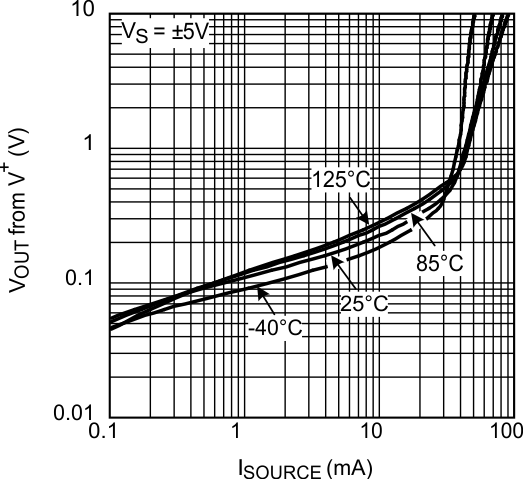Figure 3. Output Swing vs. Sourcing Current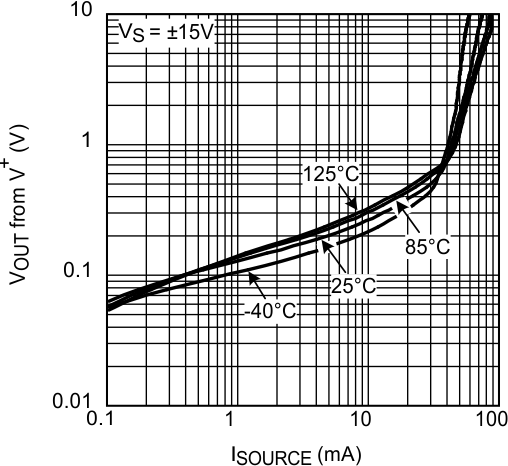Figure 5. Output Swing vs. Sourcing Current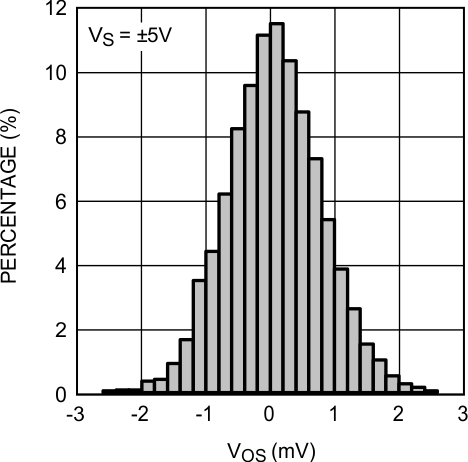Figure 7. VOS Distribution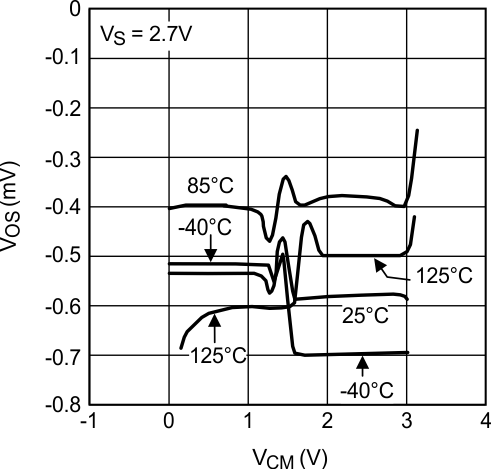Figure 9. VOS vs. VCM (Unit 2)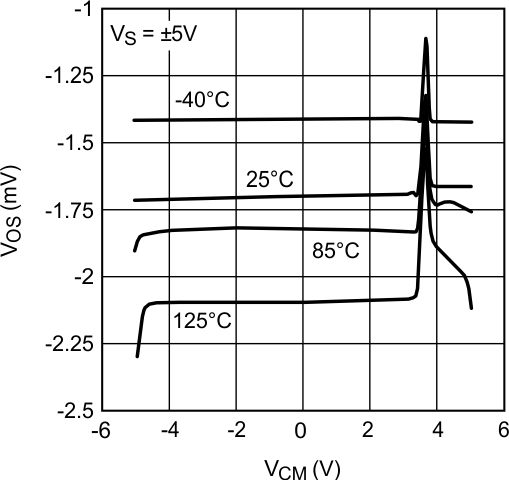Figure 11. VOS vs. VCM (Unit 1)Figure 13. VOS vs. VCM (Unit 2)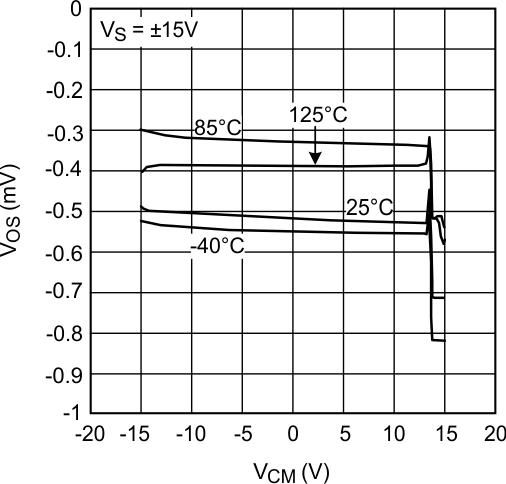Figure 15. VOS vs. VCM (Unit 2)Figure 17. VOS vs. VS (Unit 1)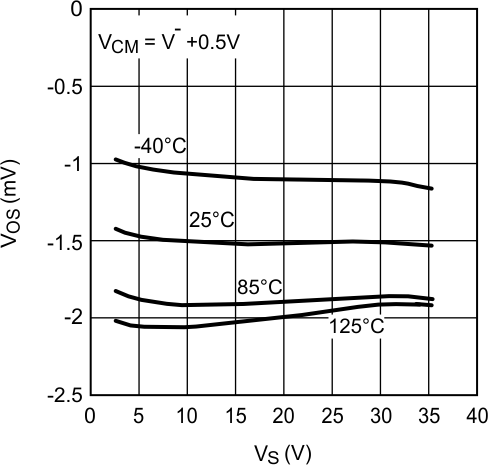Figure 19. VOS vs. VS (Unit 3)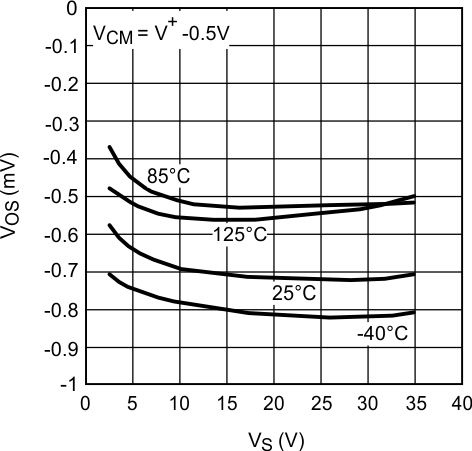Figure 21. VOS vs. VS (Unit 2)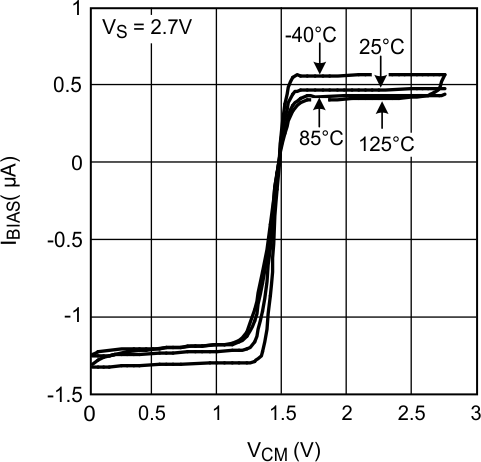Figure 23. IBIAS vs. VCM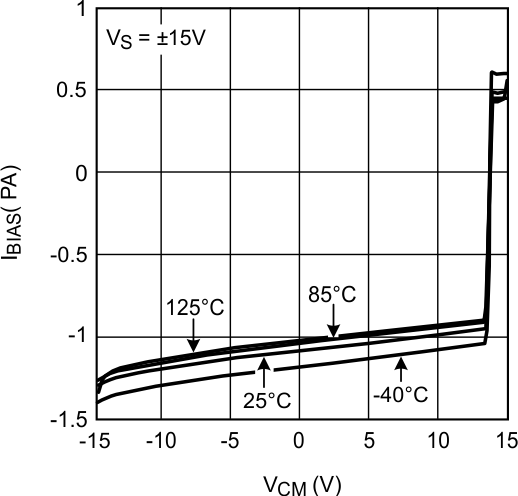Figure 25. IBIAS vs. VCM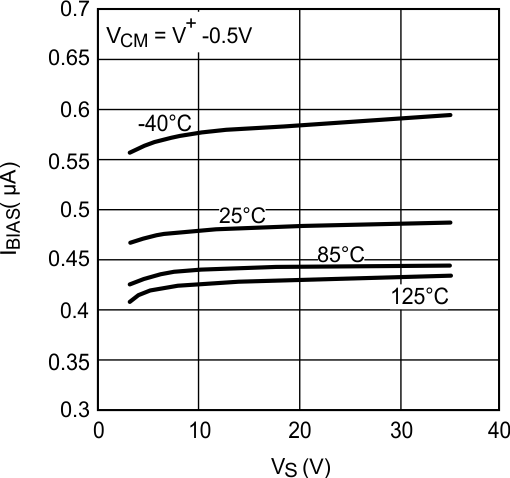Figure 27. IBIAS vs. VS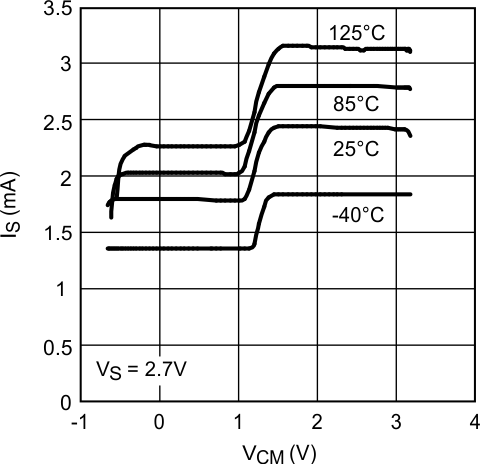Figure 29. IS vs. VCM (LM7322)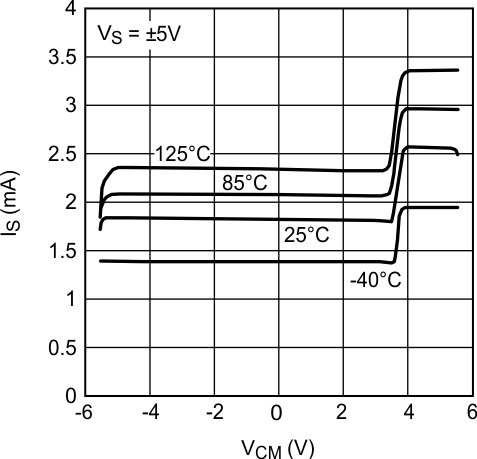Figure 31. IS vs. VCM (LM7322)Figure 33. IS vs. VCM (LM7322)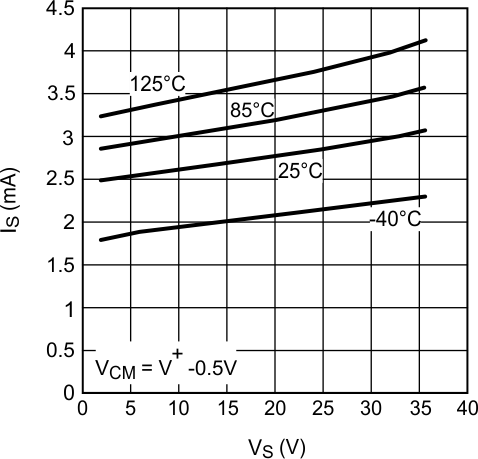Figure 35. IS vs. VS (LM7322)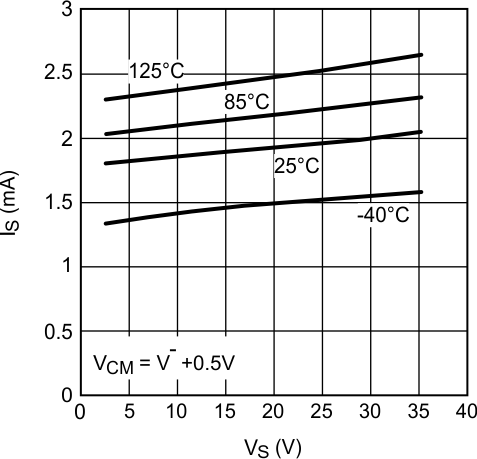Figure 37. IS vs. VS (LM7322)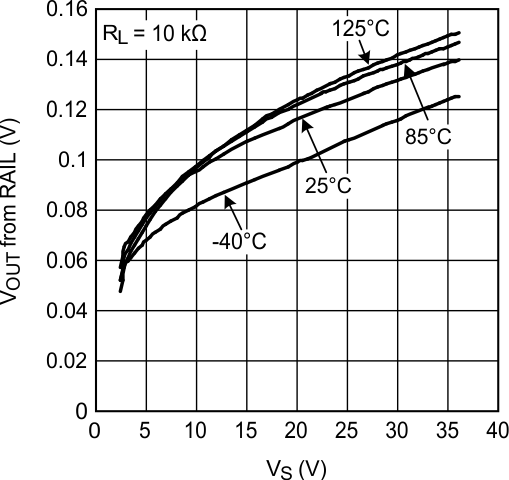Figure 39. Positive Output Swing vs. Supply Voltage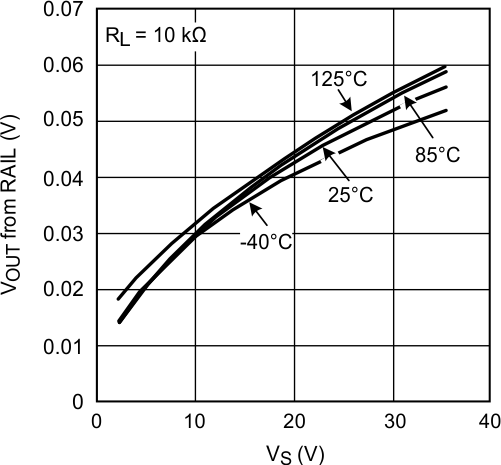Figure 41. Negative Output Swing vs. Supply Voltage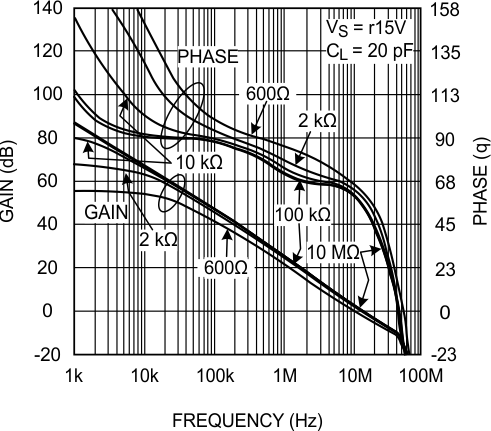Figure 43. Open-Loop Frequency Response with Various Resistive Load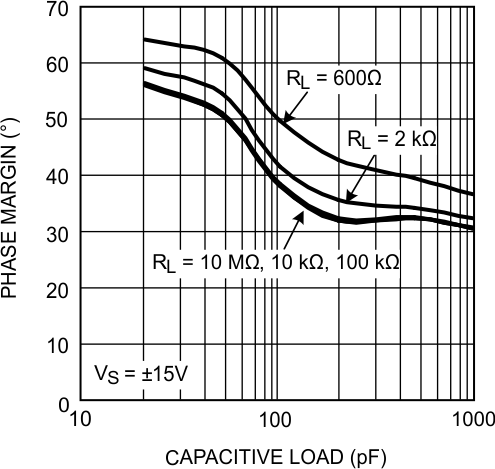Figure 45. Phase Margin vs. Capacitive Load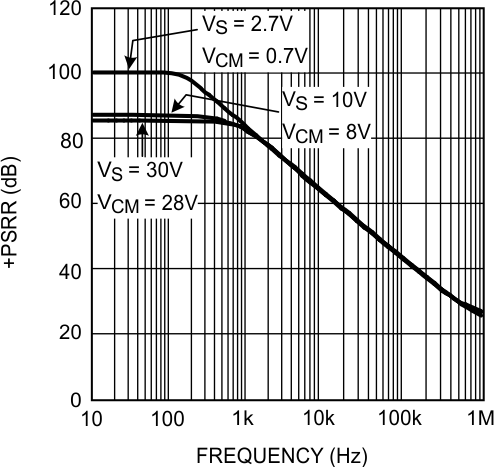Figure 47. +PSRR vs. Frequency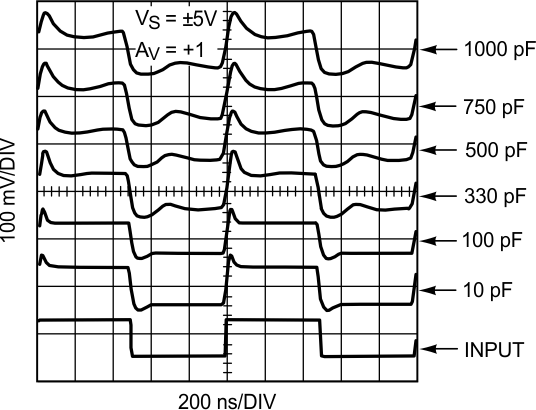Figure 49. Small Signal Step Response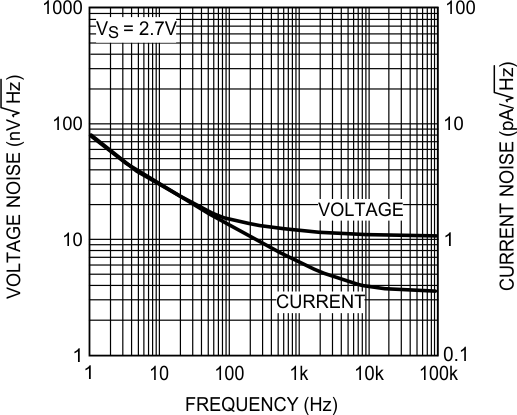Figure 51. Input Referred Noise Density vs. Frequency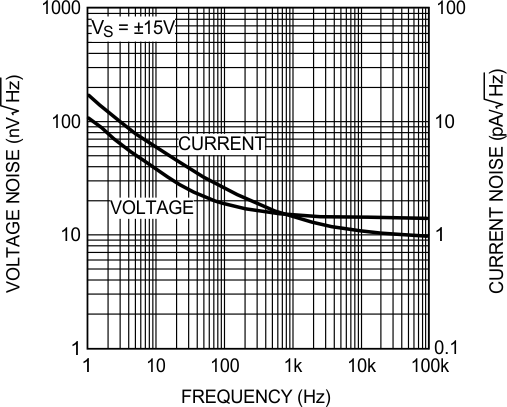Figure 53. Input Referred Noise Density vs. Frequency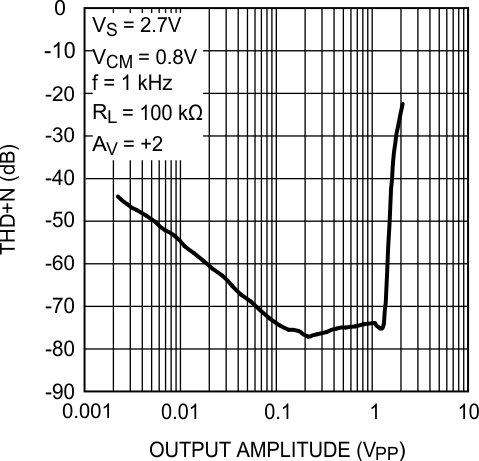Figure 55. THD+N vs. Output Amplitude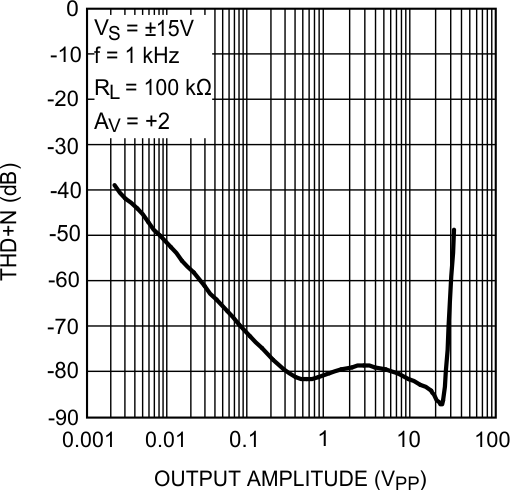Figure 57. THD+N vs. Output Amplitude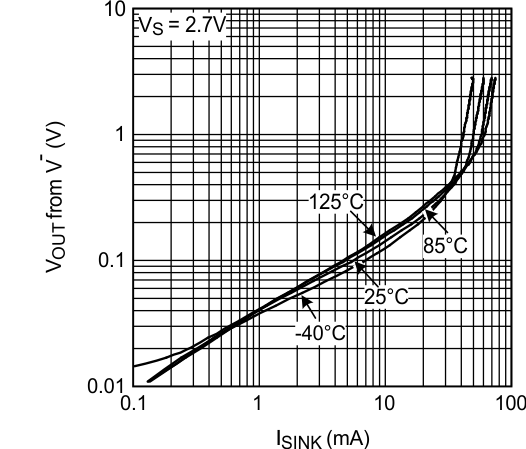Figure 2. Output Swing vs. Sinking CurrentFigure 4. Output Swing vs. Sinking Current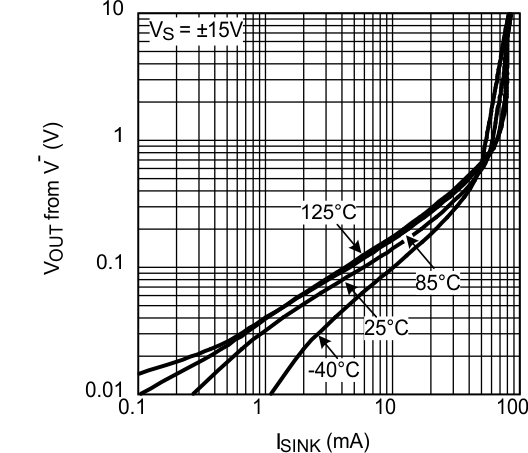Figure 6. Output Swing vs. Sinking Current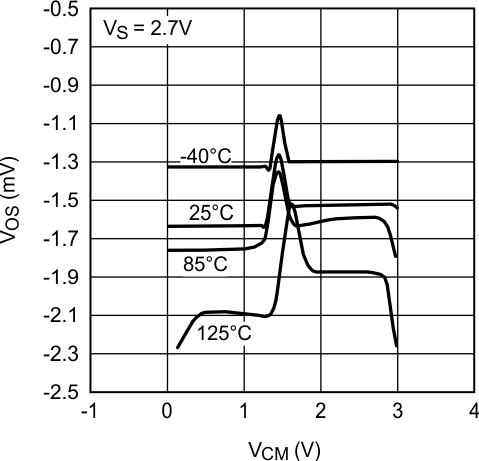Figure 8. VOS vs. VCM (Unit 1)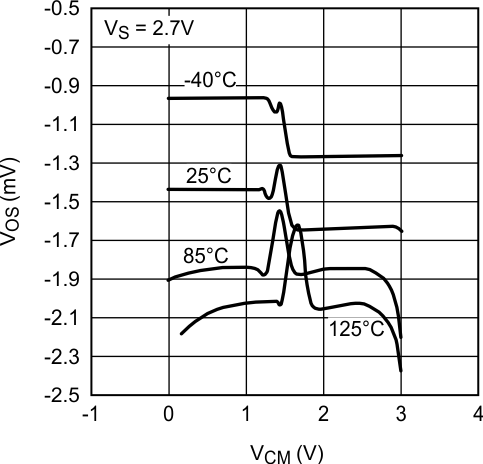Figure 10. VOS vs. VCM (Unit 3)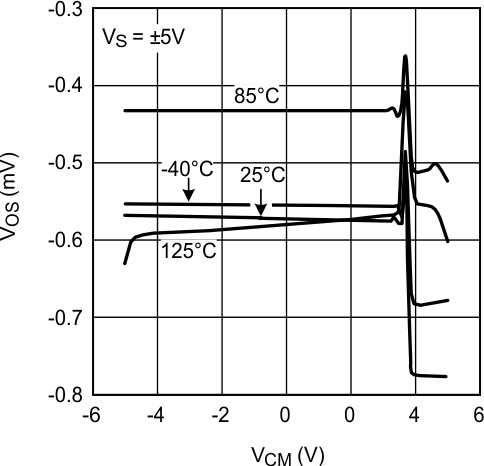Figure 12. VOS vs. VCM (Unit 2)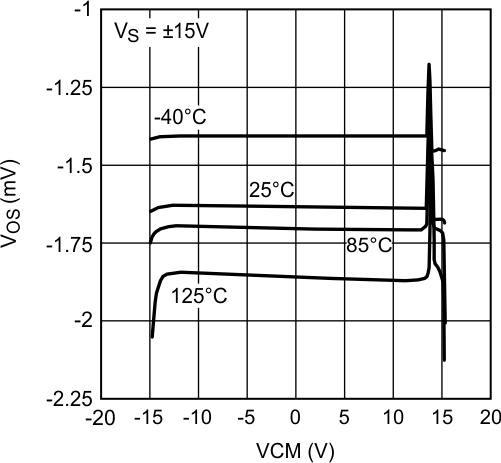Figure 14. VOS vs. VCM (Unit 1)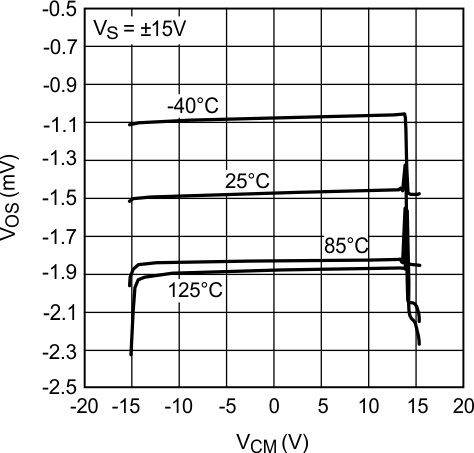Figure 16. VOS vs. VCM (Unit 3)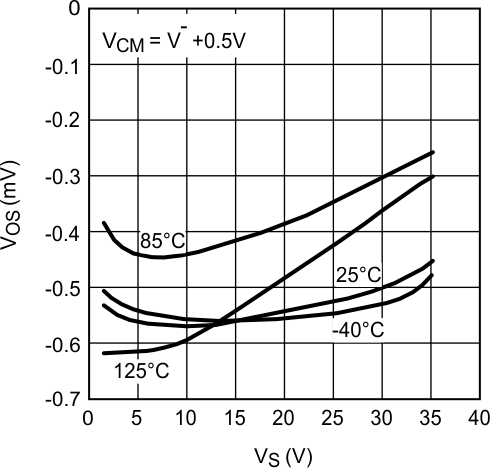Figure 18. VOS vs. VS (Unit 2)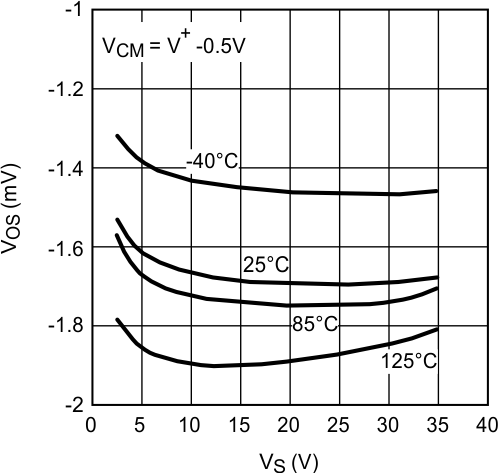Figure 20. VOS vs. VS (Unit 1)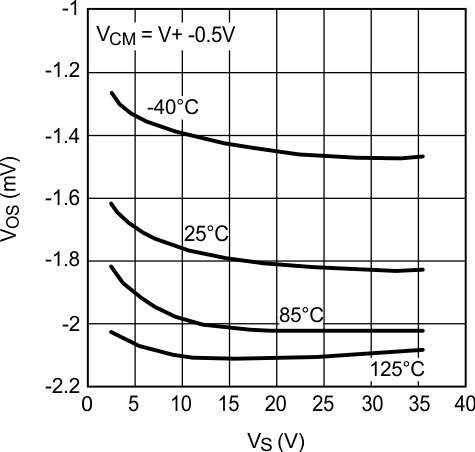Figure 22. VOS vs. VS (Unit 3)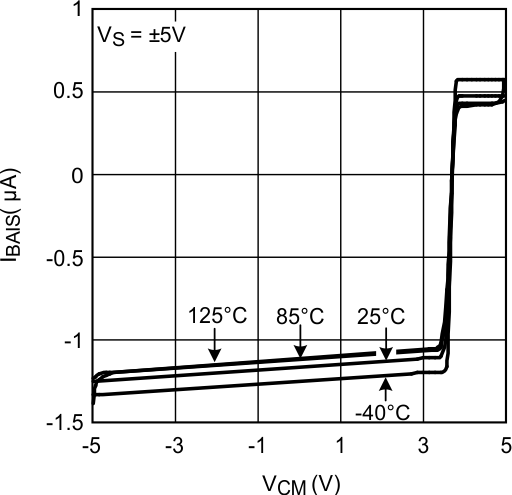Figure 24. IBIAS vs. VCM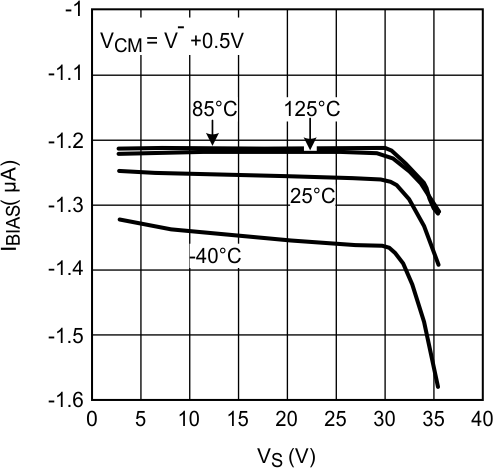Figure 26. IBIAS vs. VS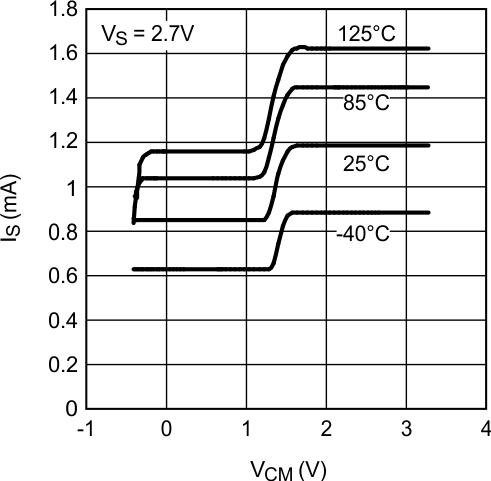Figure 28. IS vs. VCM (LM7321)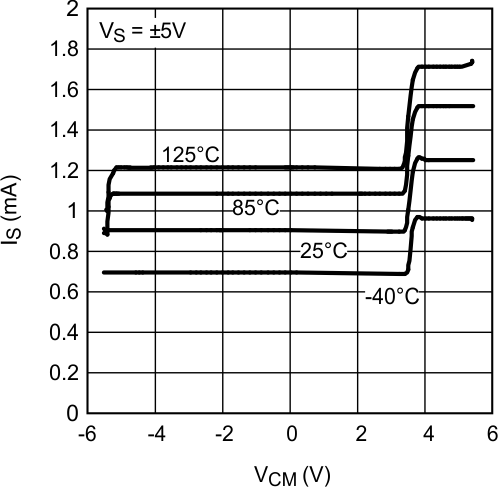Figure 30. IS vs. VCM (LM7321)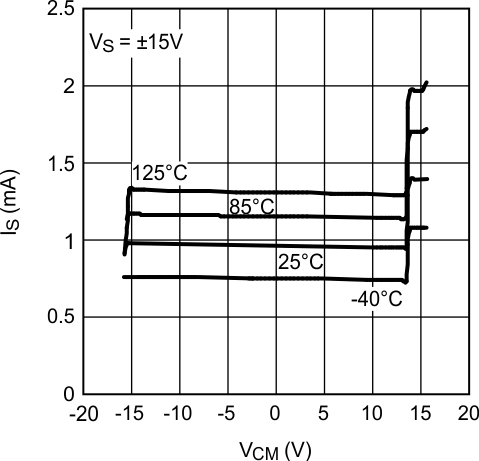Figure 32. IS vs. VCM (LM7321)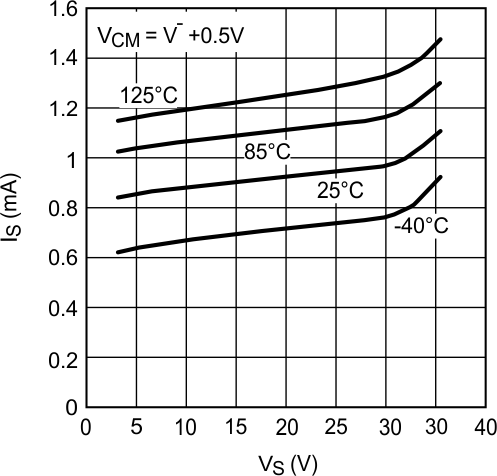Figure 34. IS vs. VS (LM7321)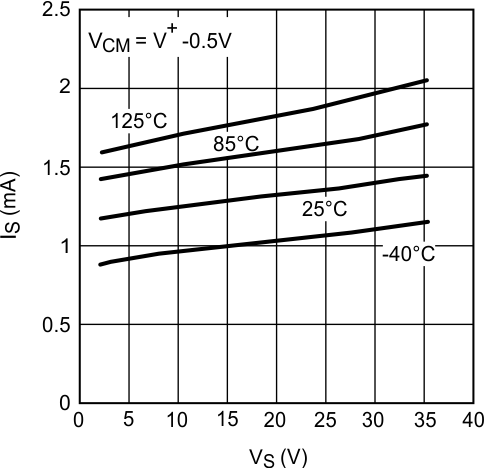Figure 36. IS vs. VS (LM7321)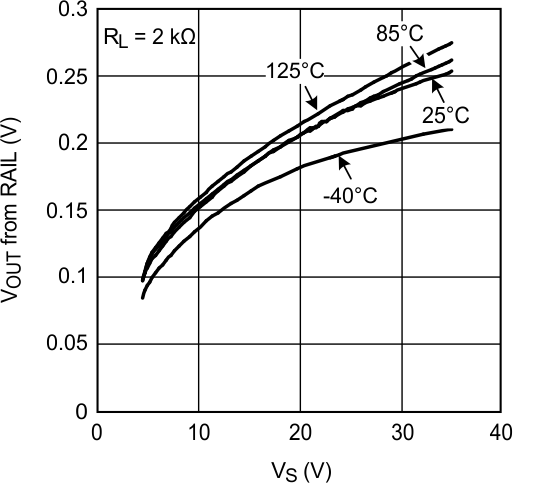Figure 38. Positive Output Swing vs. Supply VoltageFigure 40. Negative Output Swing vs. Supply Voltage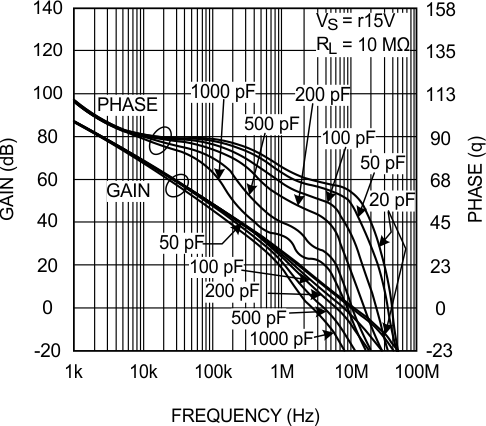Figure 42. Open-Loop Frequency Response with Various Capacitive Load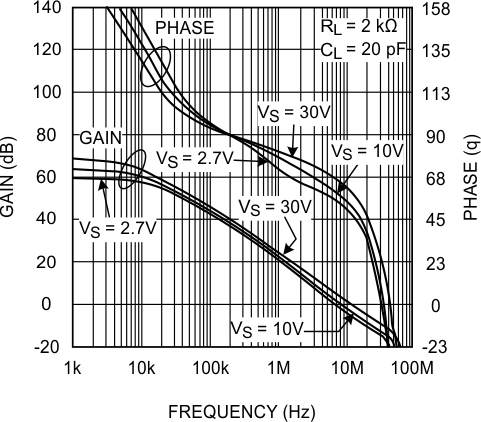Figure 44. Open-Loop Frequency Response with Various Supply Voltage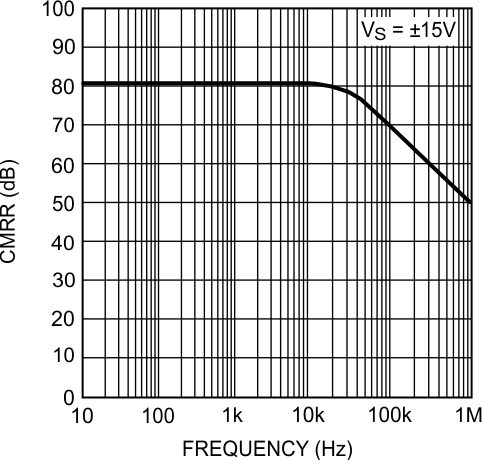Figure 46. CMRR vs. Frequency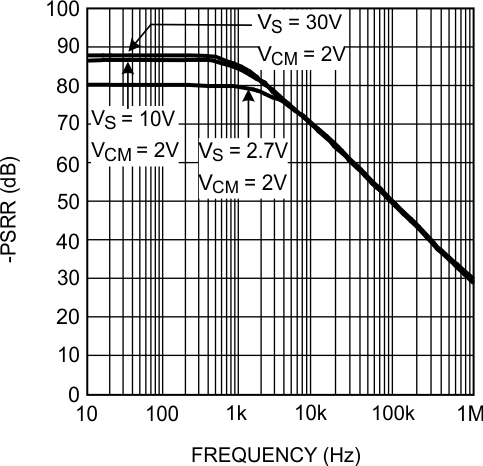Figure 48. −PSRR vs. Frequency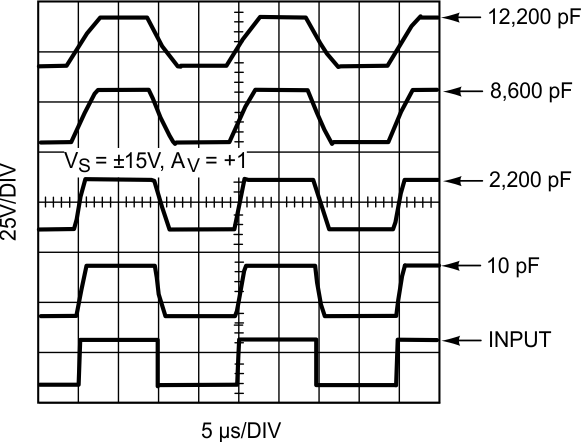Figure 50. Large Signal Step Response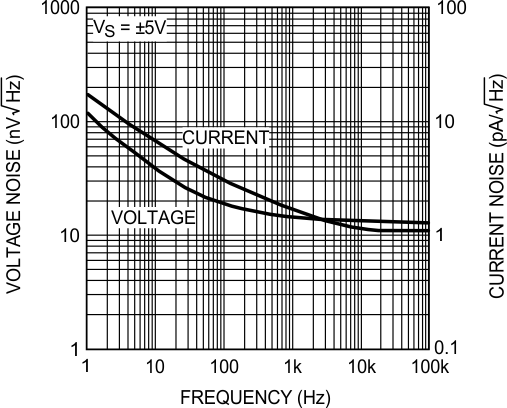Figure 52. Input Referred Noise Density vs. Frequency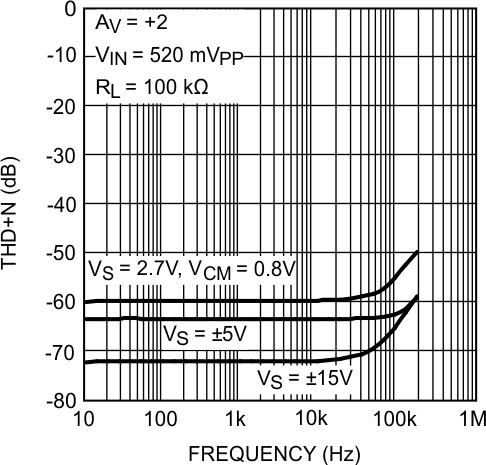Figure 54. THD+N vs. Frequency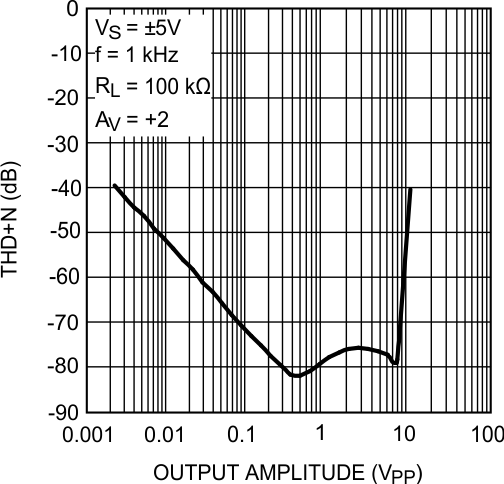Figure 56. THD+N vs. Output Amplitude